2018-12-23 15:00:54 yanghenpi 阅读数 113
• ###### Python数据挖掘实战

购买课程后，可扫码进入学习群，获取唐宇迪老师答疑 Python数据挖掘技术系列视频培训教程基于真实数据集进行案例实战，使用Python数据科学库从数据预处理开始一步步进行数据建模。对于每个案例首先进行流程解读与数据分析，建立特征工程，详细解读其中每一步原理与应用。该课程共有十一大章节，88课时，从泰坦尼克号获救预测进行数据分析作为第一章节，后边依次是用户画像、Xgboost实战、京东用户购买意向预测、Kaggle数据科学调查、房价预测、论文与BenchMark的意义、Python实现音乐推荐系统、fbprophet时间序列预测、用电敏感客户分类、数据特征。

4693 人正在学习 去看看 唐宇迪

SQLyog 导出表中数据存为csv文件

1.     选择数据库表 --> 右击属性 --> 备份/导出 --> 导出表数据作为 --> 选择cvs --> 选择下面的更改” --> 字段 --> 可变长度--> 字段终止与 -->输入逗号，(这是重点，否则导出的csv文件内容都在一列中，而不是分字段分列)
下面两个选项框取消。

2.导出csv文件后，使用UE编辑器或者记事本打开，另存为，选择编码为utf-8格式，保存。

3.打开csv文件，这样中文为正确的显示，如果不转码保存的话，为中文乱码。

SQLyog csv文件数据导入mysql表中

1.      将数据文件存为csv文件，保存的时候选择逗号(\t)作为分隔符；

2.    选择数据库表 --> 导入 --> 导入本地可使用的CSV数据 --> 从文件导入，选择刚刚的csv文件，导入完成。

3.    选择cvs --> 选择下面的更改” --> 字段 --> 可变长度--> 字段终止与 -->输入逗号，(这是重点，否则导入的csv文件内容都在一列中，而不是分字段分列)

Python Pandas处理亿级数据

• 硬件环境
• CPU：3.5 GHz Intel Core i7
• 内存：32 GB HDDR 3 1600 MHz
• 硬盘：3 TB Fusion Drive
•
• 数据分析工具
• Python：2.7.6
• Pandas：0.15.0
• IPython notebook：2.0.0
•

 Table Size Desc ServiceLogs 98,706,832 rows x 14 columns 8.77 GB 交易日志数据，每个交易会话可以有多条交易 ServiceCodes 286 rows × 8 columns 20 KB 交易分类的字典表

ipython notebook --pylab=inline

 1 ipython notebook --pylab=inline

Pandas提供了IO工具可以将大文件分块读取，测试了一下性能，完整加载9800万条数据也只需要263秒左右，还是相当不错了。

import pandas as pd reader = pd.read_csv('data/servicelogs', iterator=True) try: df = reader.get_chunk(100000000) except StopIteration: print "Iteration is stopped."

 1 2 3 4 5 6 import pandas as pd reader = pd.read_csv('data/servicelogs', iterator=True) try:     df = reader.get_chunk(100000000) except StopIteration:     print "Iteration is stopped."

 1百万条 1千万条 1亿条 ServiceLogs 1 s 17 s 263 s

loop = True chunkSize = 100000 chunks = [] while loop: try: chunk = reader.get_chunk(chunkSize) chunks.append(chunk) except StopIteration: loop = False print "Iteration is stopped." df = pd.concat(chunks, ignore_index=True)

 1 2 3 4 5 6 7 8 9 10 11 loop = True chunkSize = 100000 chunks = [] while loop:     try:         chunk = reader.get_chunk(chunkSize)         chunks.append(chunk)     except StopIteration:         loop = False         print "Iteration is stopped." df = pd.concat(chunks, ignore_index=True)

 Chunk Size Read Time (s) Total Time (s) Performance 100,000 224.418173 261.358521 200,000 232.076794 256.674154 1,000,000 213.128481 234.934142 √ √ 2,000,000 208.410618 230.006299 √ √ √ 5,000,000 209.460829 230.939319 √ √ √ 10,000,000 207.082081 228.135672 √ √ √ √ 20,000,000 209.628596 230.775713 √ √ √ 50,000,000 222.910643 242.405967 100,000,000 263.574246 263.574246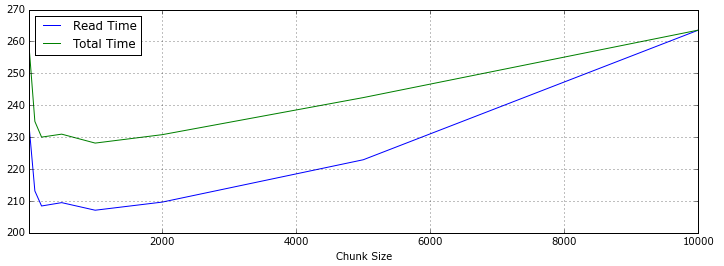## 数据清洗

Pandas提供了 DataFrame.describe 方法查看数据摘要，包括数据查看（默认共输出首尾60行数据）和行列统计。由于源数据通常包含一些空值甚至空列，会影响数据分析的时间和效率，在预览了数据摘要后，需要对这些无效数据进行处理。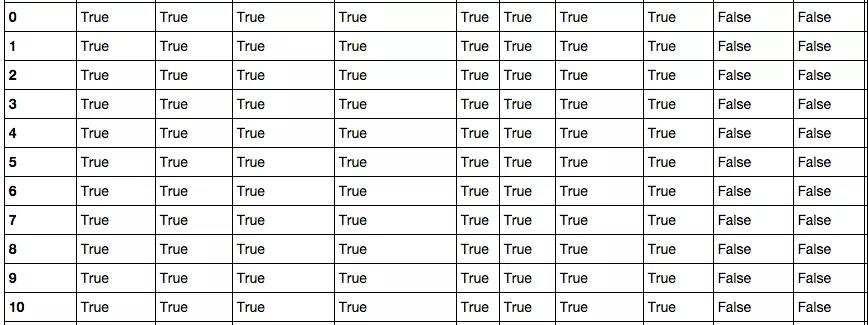Pandas的非空计算速度很快，9800万数据也只需要28.7秒。得到初步信息之后，可以对表中空列进行移除操作。尝试了按列名依次计算获取非空列，和 DataFrame.dropna() 两种方式，时间分别为367.0秒和345.3秒，但检查时发现 dropna() 之后所有的行都没有了，查了Pandas手册，原来不加参数的情况下， dropna() 会移除所有包含空值的行。如果只想移除全部为空值的列，需要加上 axis how 两个参数：

df.dropna(axis=1, how='all')

 1 df.dropna(axis=1, how='all')

## 数据处理

df['Name'] = df['Name'].astype(np.datetime64)

 1 df['Name'] = df['Name'].astype(np.datetime64)

df.groupby(['NO','TIME','SVID']).count() # 分组 fullData = pd.merge(df, trancodeData)[['NO','SVID','TIME','CLASS','TYPE']] # 连接 actions = fullData.pivot_table('SVID', columns='TYPE', aggfunc='count') # 透视表

 1 2 3 df.groupby(['NO','TIME','SVID']).count() # 分组 fullData = pd.merge(df, trancodeData)[['NO','SVID','TIME','CLASS','TYPE']] # 连接 actions = fullData.pivot_table('SVID', columns='TYPE', aggfunc='count') # 透视表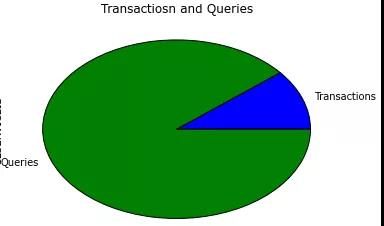total_actions = fullData.pivot_table('SVID', index='TIME', columns='TYPE', aggfunc='count') total_actions.plot(subplots=False, figsize=(18,6), kind='area')

 1 2 total_actions = fullData.pivot_table('SVID', index='TIME', columns='TYPE', aggfunc='count') total_actions.plot(subplots=False, figsize=(18,6), kind='area')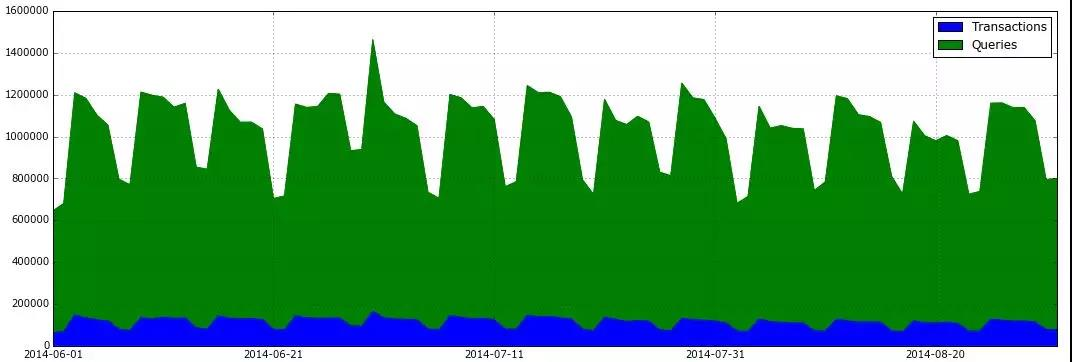除此之外，Pandas提供的DataFrame查询统计功能速度表现也非常优秀，7秒以内就可以查询生成所有类型为交易的数据子表：

tranData = fullData[fullData['Type'] == 'Transaction']

 1 tranData = fullData[fullData['Type'] == 'Transaction']

python 大数据处理案例 相关内容

2017-06-25 22:41:00 diaozhu1028 阅读数 7
• ###### Python数据挖掘实战

购买课程后，可扫码进入学习群，获取唐宇迪老师答疑 Python数据挖掘技术系列视频培训教程基于真实数据集进行案例实战，使用Python数据科学库从数据预处理开始一步步进行数据建模。对于每个案例首先进行流程解读与数据分析，建立特征工程，详细解读其中每一步原理与应用。该课程共有十一大章节，88课时，从泰坦尼克号获救预测进行数据分析作为第一章节，后边依次是用户画像、Xgboost实战、京东用户购买意向预测、Kaggle数据科学调查、房价预测、论文与BenchMark的意义、Python实现音乐推荐系统、fbprophet时间序列预测、用电敏感客户分类、数据特征。

4693 人正在学习 去看看 唐宇迪lubridate包拆解时间 | POSIXlt

https://www.kaggle.com/c/bike-sharing-demand1.png

``````library(lubridate)
library(randomForest)

setwd("E:")

``````test\$registered=0
test\$casual=0
test\$count=0
data<-rbind(train,test)``````

``````data\$hour1<-substr(data\$datetime,12,13)
table(data\$hour1)``````6-hour1.png5-hour-regestered.png5-hour-casual.png4-boxplot-day.pngcor.png

``````train\$hour1<-as.integer(train\$hour1)
d<-rpart(registered~hour1,data=train)
rpart.plot(d)``````3-raprt-hour1.png

``````train\$hour1<-as.integer(train\$hour1)
data\$dp_reg=0
data\$dp_reg[data\$hour1<7.5]=1
data\$dp_reg[data\$hour1>=22]=2
data\$dp_reg[data\$hour1>=9.5 & data\$hour1<18]=3
data\$dp_reg[data\$hour1>=7.5 & data\$hour1<18]=4
data\$dp_reg[data\$hour1>=8.5 & data\$hour1<18]=5
data\$dp_reg[data\$hour1>=20 & data\$hour1<20]=6
data\$dp_reg[data\$hour1>=18 & data\$hour1<20]=7``````3-raprt-temp.png

``````data\$year_part=0
data\$month<-month(data\$datatime)
data\$year_part[data\$year=='2011']=1
data\$year_part[data\$year=='2011' & data\$month>3]=2
data\$year_part[data\$year=='2011' & data\$month>6]=3
data\$year_part[data\$year=='2011' & data\$month>9]=4``````
``````data\$day_type=""
data\$day_type[data\$holiday==0 & data\$workingday==0]="weekend"
data\$day_type[data\$holiday==1]="holiday"
data\$day_type[data\$holiday==0 & data\$workingday==1]="working day"
data\$weekend=0
data\$weekend[data\$day=="Sunday"|data\$day=="Saturday"]=1``````

ntree指定随机森林所包含的决策树数目，默认为500，通常在性能允许的情况下越大越好；
mtry指定节点中用于二叉树的变量个数，默认情况下数据集变量个数的二次方根（分类模型）或三分之一（预测模型）。一般是需要进行人为的逐次挑选，确定最佳的m值—摘自datacruiser笔记。这里我主要学习，所以虽然有10000多数据集，但也只定了500。就这500我的小电脑也跑了半天。

``````train<-data
set.seed(1234)
train\$logreg<-log(train\$registered+1)
test\$logcas<-log(train\$casual+1)

fit1<-randomForest(logreg~hour1+workingday+day+holiday+day_type+temp_reg+humidity+atemp+windspeed+season+weather+dp_reg+weekend+year+year_part,train,importance=TRUE,ntree=250)

pred1<-predict(fit1,train)
train\$logreg<-pred1``````

``````train\$registered<-exp(train\$logreg)-1
train\$casual<-exp(train\$logcas)-1
train\$count<-test\$casual+train\$registered``````

``````train2<-train[as.integer(day(data\$datetime))>=20,]
submit_final<-data.frame(datetime=test\$datetime,count=test\$count)
write.csv(submit_final,"submit_final.csv",row.names=F)``````

github代码加群

626062078，我们一起学Python！

python 大数据处理案例 相关内容

2019-09-21 23:24:46 dafeidouzi 阅读数 127
• ###### Python数据挖掘实战

购买课程后，可扫码进入学习群，获取唐宇迪老师答疑 Python数据挖掘技术系列视频培训教程基于真实数据集进行案例实战，使用Python数据科学库从数据预处理开始一步步进行数据建模。对于每个案例首先进行流程解读与数据分析，建立特征工程，详细解读其中每一步原理与应用。该课程共有十一大章节，88课时，从泰坦尼克号获救预测进行数据分析作为第一章节，后边依次是用户画像、Xgboost实战、京东用户购买意向预测、Kaggle数据科学调查、房价预测、论文与BenchMark的意义、Python实现音乐推荐系统、fbprophet时间序列预测、用电敏感客户分类、数据特征。

4693 人正在学习 去看看 唐宇迪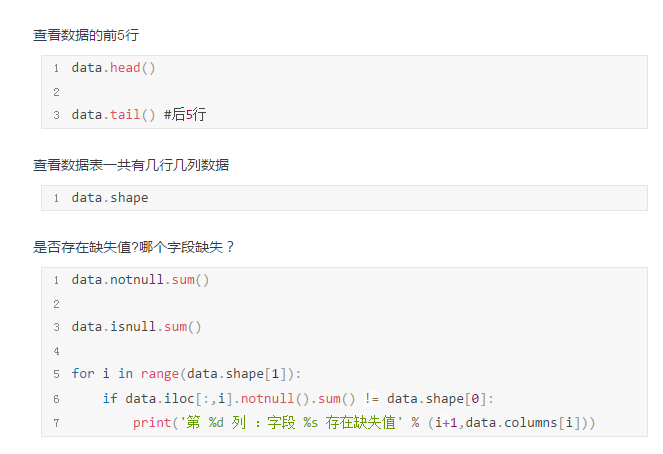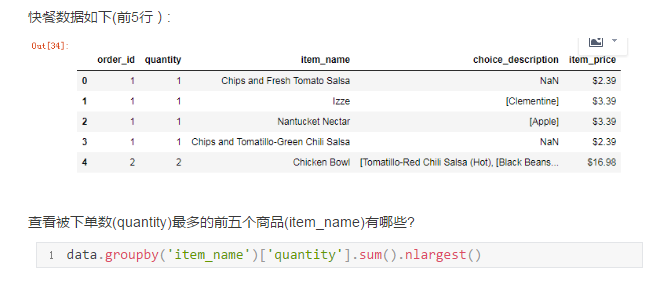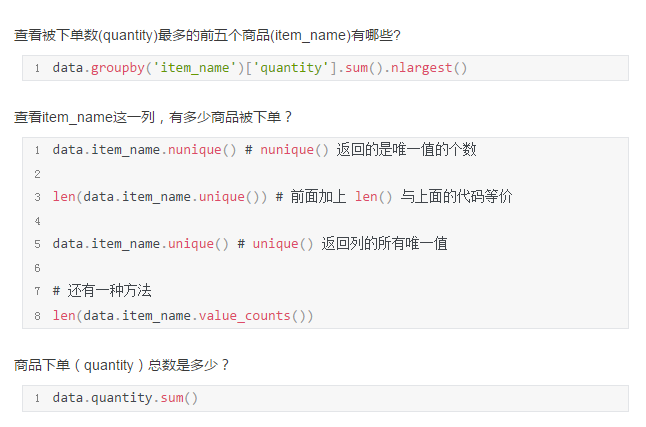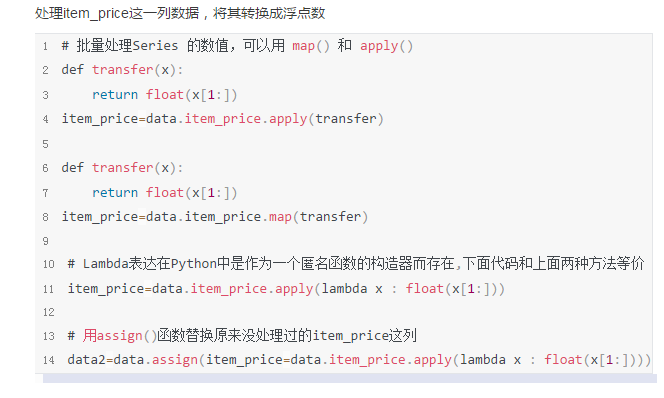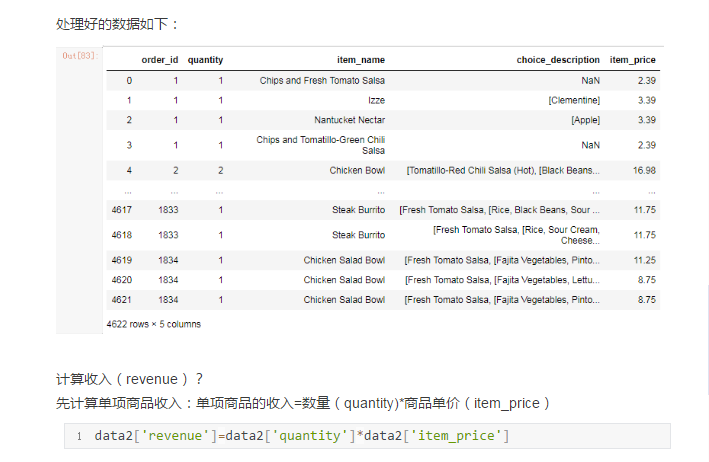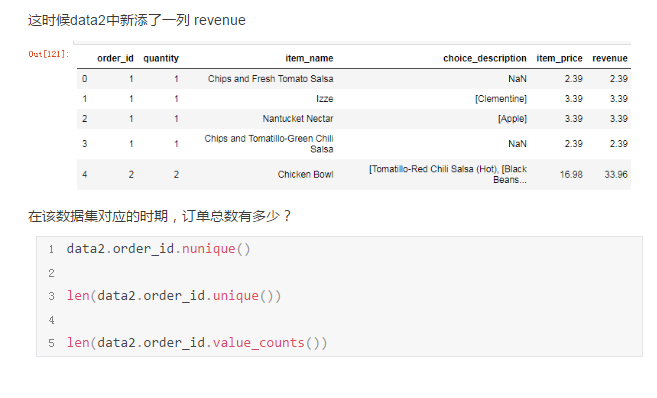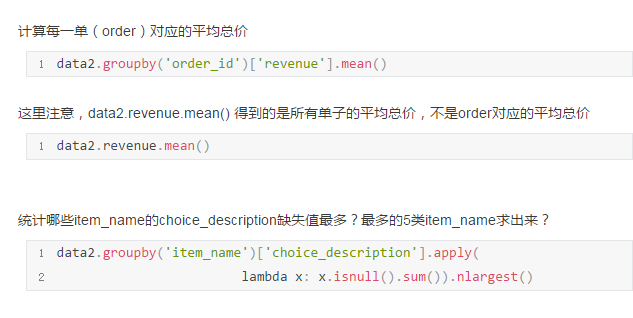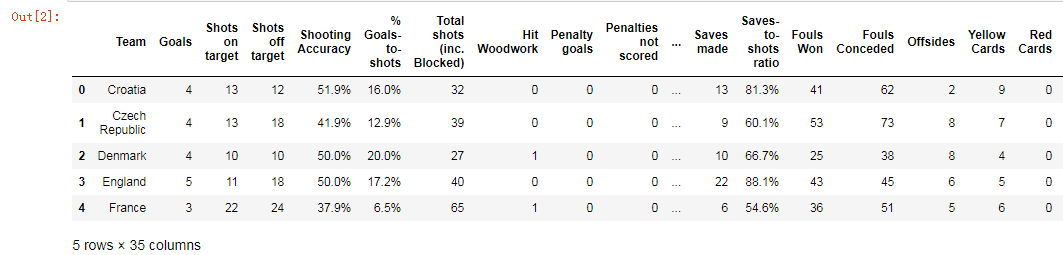``````data.info()

data.describe()
``````

``````for i in range(data.shape):
if data.iloc[:,i].notnull().sum() != data.shape:
print('第%d列：字段%s 存在缺失值'%(i+1,data.columns[i]))``````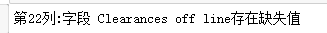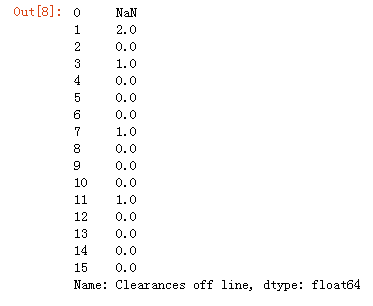``data['Clearances off line'].value_counts()``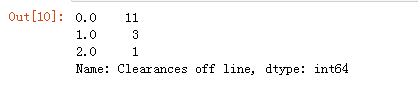``````mode=data['Clearances off line'].mode()
data['Clearances off line']=data['Clearances off line'].fillna(mode)``````

``data.Team.count()``

``discipline=data[['Team','Yellow Cards','Red Cards']]``

``discipline.sort_values(by=['Red Cards','Yellow Cards'])``

``data['Yellow Cards'].mean()``

``data[data['Goals']>6]``

``data['Shooting Accuracy'].[data.Team.isin(['England','ltaly','Russia'])]``

python 大数据处理案例 相关内容

2018-06-12 18:11:12 jianghuming 阅读数 404
• ###### Python数据挖掘实战

购买课程后，可扫码进入学习群，获取唐宇迪老师答疑 Python数据挖掘技术系列视频培训教程基于真实数据集进行案例实战，使用Python数据科学库从数据预处理开始一步步进行数据建模。对于每个案例首先进行流程解读与数据分析，建立特征工程，详细解读其中每一步原理与应用。该课程共有十一大章节，88课时，从泰坦尼克号获救预测进行数据分析作为第一章节，后边依次是用户画像、Xgboost实战、京东用户购买意向预测、Kaggle数据科学调查、房价预测、论文与BenchMark的意义、Python实现音乐推荐系统、fbprophet时间序列预测、用电敏感客户分类、数据特征。

4693 人正在学习 去看看 唐宇迪

Python大数据分析_开篇

﻿﻿

python 大数据处理案例 相关内容

2018-05-24 19:50:48 qq_39343820 阅读数 165
• ###### Python数据挖掘实战

购买课程后，可扫码进入学习群，获取唐宇迪老师答疑 Python数据挖掘技术系列视频培训教程基于真实数据集进行案例实战，使用Python数据科学库从数据预处理开始一步步进行数据建模。对于每个案例首先进行流程解读与数据分析，建立特征工程，详细解读其中每一步原理与应用。该课程共有十一大章节，88课时，从泰坦尼克号获救预测进行数据分析作为第一章节，后边依次是用户画像、Xgboost实战、京东用户购买意向预测、Kaggle数据科学调查、房价预测、论文与BenchMark的意义、Python实现音乐推荐系统、fbprophet时间序列预测、用电敏感客户分类、数据特征。

4693 人正在学习 去看看 唐宇迪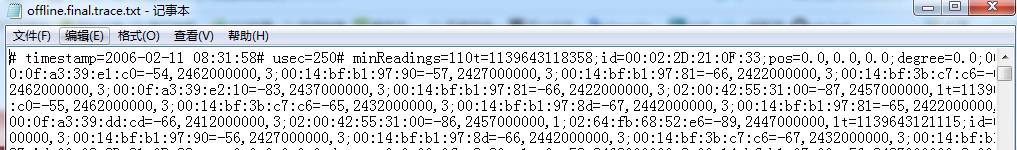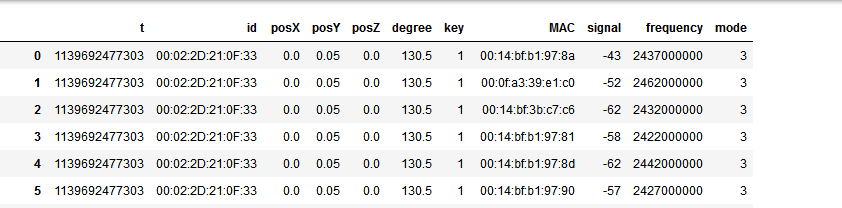```with open('G:\Positioning\online.final.trace.txt','r') as r:
with open('G:\Positioning\online.final.trace.txt','w') as w:
for l in lines:
if '#' not in l:
w.write(l)  #去除带有‘#’字符的行```

```f = open('G:\Positioning\Positioning.txt')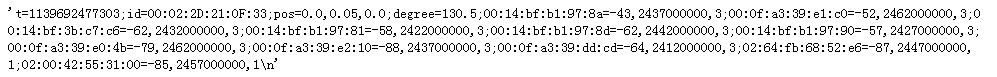```import re
tokens = re.split('[=;,]',data) #正则表达式，分离各个元素，根据‘=’，‘;’，‘,’这三个字符对数据进行分离出来放在一个列表中```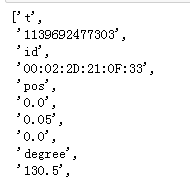```import pandas as pd
import numpy as np
from pandas import Series, DataFrame #引入约定
columns1 = [tokens,tokens,'posX','posY','posZ',tokens] #得到第一部分数据的列表名
data1 = [tokens,tokens,tokens,tokens,tokens,tokens] #得到第一部分的数据
s = Series(data1, index = columns1) #第一部分的Series
df1 = DataFrame(s).T #第一部分数据的DataFrame```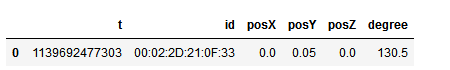```a = tokens[10:] #第二部分的数据
columns2 = ['MAC','signal','frequency','mode']  #第二部分数据的列名
def chunks(s, step):
return [s[i:i+step] for i in  range(0, len(s), step)] #函数：遍历列表，取n个元素为一个新元素
data2 = chunks(a, 4) #每四个一组
df2 = DataFrame(data2, columns = columns2 ) #第二部分数据的DataFrame```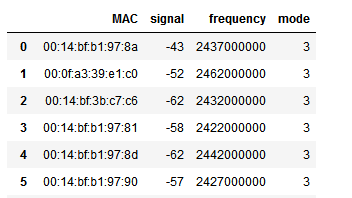```df1['key'] ='1' #增添一个公共键
df2['key'] ='1'
df=pd.merge(df1,df2) #合并```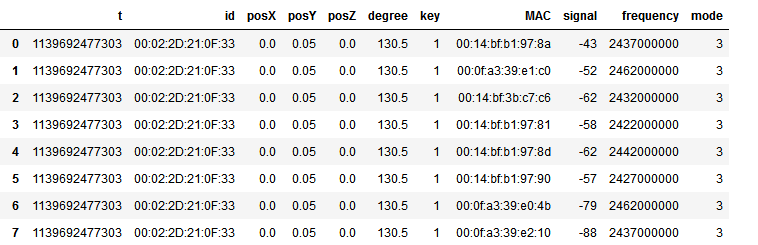```with open('G:\Positioning\online.final.trace.txt','r') as r:
with open('G:\Positioning\online.final.trace.txt','w') as w:
for l in lines:
if '#' not in l:
w.write(l)
#删除含有#的行```

```import pandas as pd
import numpy as np
from pandas import Series, DataFrame

def function(x): #编写函数
import re
import pandas as pd
import numpy as np
from pandas import Series, DataFrame
tokens = re.split('[=;,]',x) #分离各个元素

columns1 = [tokens,tokens,'posX','posY','posZ',tokens] #得到第一部分的列表名
data1 = [tokens,tokens,tokens,tokens,tokens,tokens] #得到第一部分的数据
s = Series(data1, index = columns1) #第一部分的Series
df1 = DataFrame(s).T #第一部分的DataFrame

a = tokens[10:] #第二部分的数据
columns2 = ['MAC','signal','frequency','mode']
def chunks(s, step):
return [s[i:i+step] for i in  range(0, len(s), step)]
data2 = chunks(a, 4) #每四个一组
df2 = DataFrame(data2, columns = columns2 )

df1['key'] ='1' #增添公共键
df2['key'] ='1'

df=pd.merge(df1,df2) #合并

return df```

```f = open('G:\Positioning\online.final.trace.txt')
lines = [line.strip('\n') for line in f] #去掉换行符
frames=[] #先设置空的列表
for i in lines:
frames.append(function(i)) #将lines中每个元素进行应用函数后添加到列表中
df = pd.concat(frames, ignore_index = True) #无视索引，重新编号```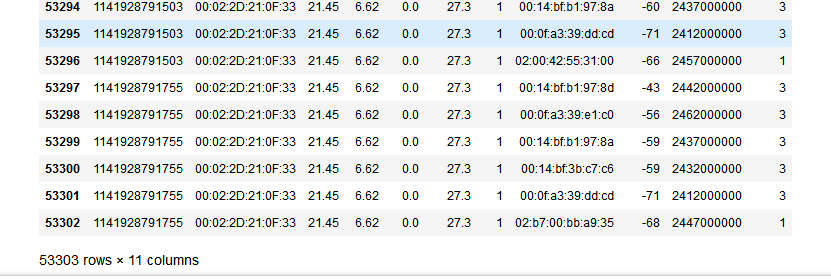python 大数据处理案例 相关内容